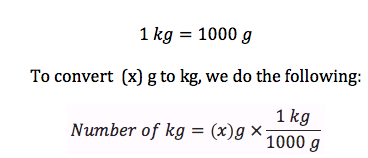The mass of a moon rock is 3.5 kilograms. What is the mass of the moon rock in grams?

Dec 30, 2016

$3.5 \times {10}^{3} g$

Explanation:

In order to figure out the number of grams in 3.5 kilograms, we're going to use dimensional analysis (method that allows you to convert from one unit of measurement to another by cancelling out unwanted units) and the relationship below:For questions like this I use the following technique:

$\text{Quantity Given" xx "Conversion Factor" = "Quantity Sought}$

color(pink)("Quantity Given:" 3.5 kilograms

color(green)("Conversion Factor:" 1000

color(red)("Quantity Sought:" grams

Let's plug in our values:

3.5cancel"kg"xx(1000g)/(1cancel"kg") = $3.5 \times {10}^{3} g$